## RD Sharma Class 8 Solutions Chapter 1 Rational Numbers Ex 1.3

These Solutions are part of RD Sharma Class 8 Solutions. Here we have given RD Sharma Class 8 Solutions Chapter 1 Rational Numbers Ex 1.3

Other Exercises

Question 1.
Subtract the first rational number from the second in each of the following :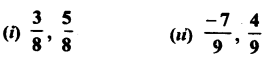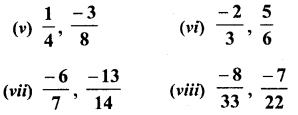Solution: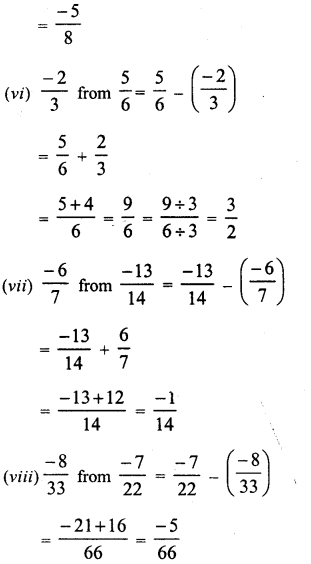Question 2.
Evaluate each of the following :Solution: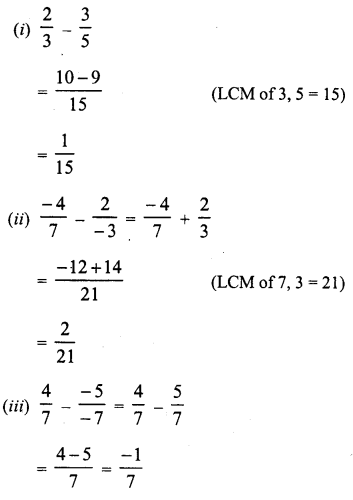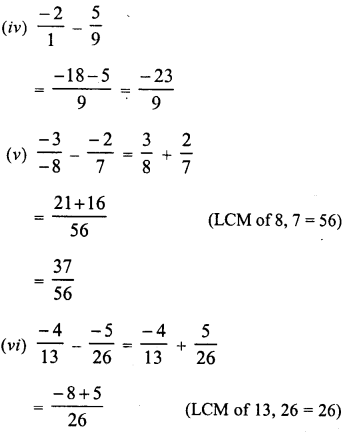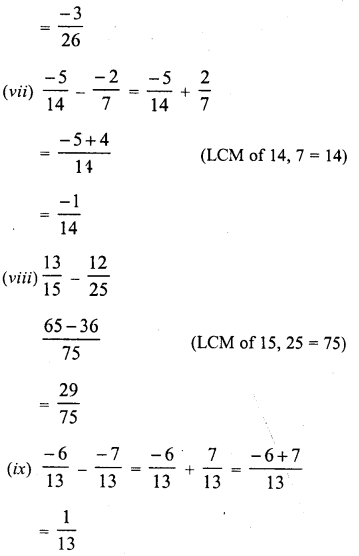Question 3.
The sum of two numbers is $$\frac { 5 }{ 9 }$$. If one of the numbers is $$\frac { 1 }{ 3 }$$, find the other.
Solution: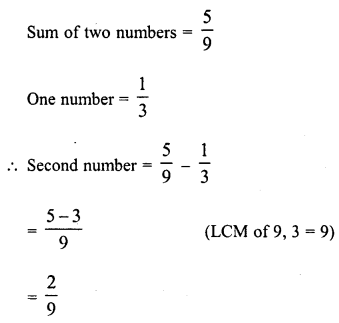Question 4.
The sum of two numbers is $$\frac { -1 }{ 3 }$$. If one of the numbers is $$\frac { -12 }{ 3 }$$, find the other.
Solution: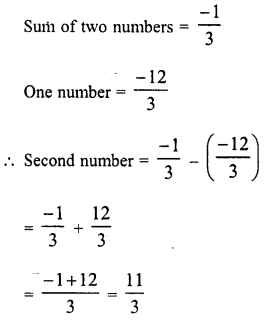Question 5.
The sum of two numbers is $$\frac { -4 }{ 3 }$$. If one of the number is -5, find the
Solution:Question 6.
The sum of two rational numbers is -8. If one of the numbers is $$\frac { -15 }{ 7 }$$ find the other.
Solution: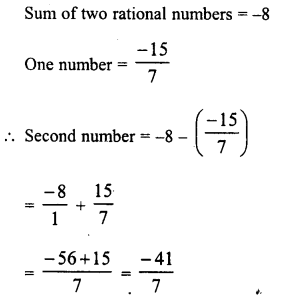Question 7.
What should be added to so as to $$\frac { -7 }{ 8 }$$ get $$\frac { 5 }{ 9 }$$ ?
Solution: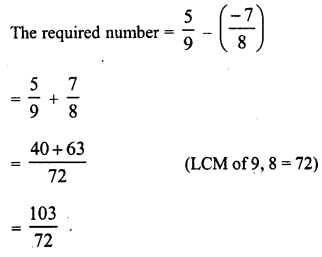Question 8.
What number should be added to $$\frac { -5 }{ 11 }$$ so as to get $$\frac { 26 }{ 3 }$$ ?
Solution: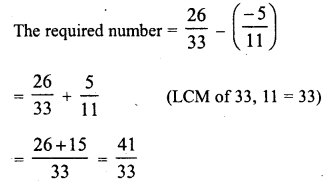Question 9.
What number should be added to $$\frac { -5 }{ 7 }$$ to get $$\frac { -2 }{ 3 }$$ ?
Solution: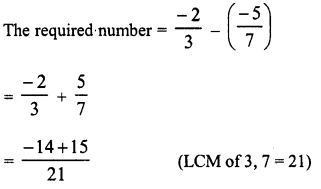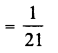Question 10.
What number should be subtracted from $$\frac { -5 }{ 3 }$$ to get $$\frac { 5 }{ 6 }$$ ?
Solution:Question 11.
What number should be subtracted from $$\frac { 3 }{ 7 }$$ to get $$\frac { 5 }{ 4 }$$ ?
Solution: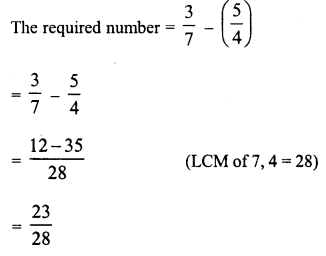Question 12.
What should be added to $$\left( \frac { 2 }{ 3 } +\frac { 3 }{ 5 } \right)$$ to get $$\frac { -12 }{ 15 }$$ ?
Solution: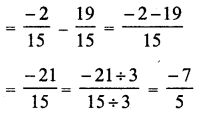Question 13.
What should be added to $$\left( \frac { 1 }{ 2 } +\frac { 1 }{ 3 } +\frac { 1 }{ 5 } \right)$$ to get 3 ?
Solution:Question 14.
What should be subtracted from $$\left( \frac { 3 }{ 4 } -\frac { 2 }{ 3 } \right)$$ to get $$\frac { -1 }{ 6 }$$ ?
Solution:Question 15.
Fill in the blanks :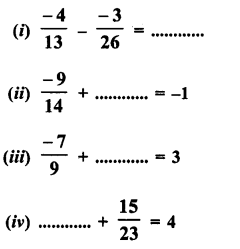Solution: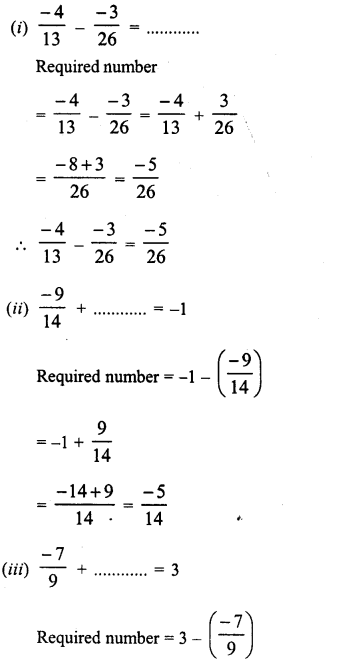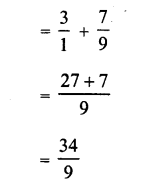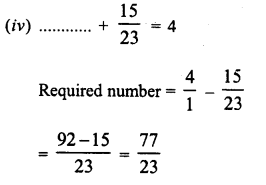Hope given RD Sharma Class 8 Solutions Chapter 1 Rational Numbers Ex 1.3 are helpful to complete your math homework.

If you have any doubts, please comment below. Learn Insta try to provide online math tutoring for you.